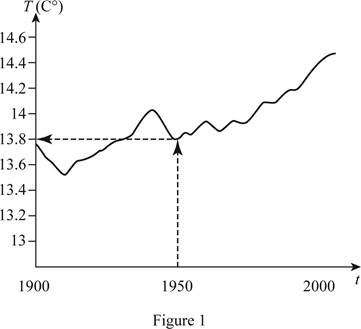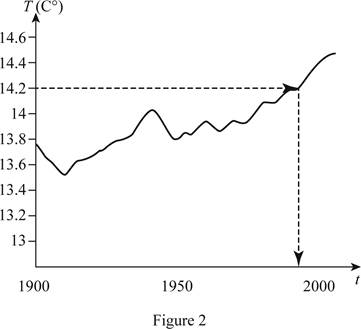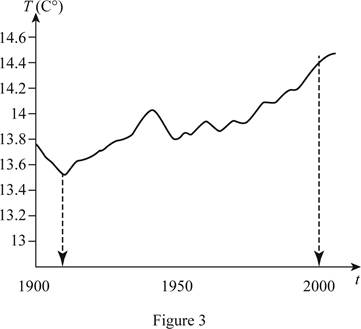# To estimate: The global average temperature in the year 1950.### Single Variable Calculus: Concepts...

4th Edition
James Stewart
Publisher: Cengage Learning
ISBN: 9781337687805### Single Variable Calculus: Concepts...

4th Edition
James Stewart
Publisher: Cengage Learning
ISBN: 9781337687805

#### Solutions

Chapter 1.1, Problem 9E

(a)

To determine

## To estimate: The global average temperature in the year 1950.

Expert Solution

The global average temperature in 1950 is 13.8°C.

### Explanation of Solution

During the 20th century, the global average temperature in the graph, the x-axis represents the period (t) between 1900 and 2000, and the y-axis represents the global average temperature (T) in degree Celsius.

Draw the vertical line from the year 1950 to meet the graph of global average temperature during the 20th century. And then, draw the horizontal line towards the y-axis as shown below in Figure 1.From Figure 1, it is found that the temperature in 1950 is below 14°C and approximate to 13.8°C. Therefore, the temperature was about13.8°C.

(b)

To determine

### To estimate: The year when the average temperature was 14.2°C.

Expert Solution

The average temperature was nearly 14.2°C in the year 1990.

### Explanation of Solution

Draw the horizontal line from 14.2°C to meet the graph of global average temperature during the 20th century. And then, draw the vertical line until it meets the x-axis, which is shown below in Figure 2.From Figure 2, it is found that the average temperature was 14.2°C in the year 1990.

(c)

To determine

### To estimate: The year in which the temperature was too high and too low.

Expert Solution

The temperature was too low in the year 1910 and it was too high in the year 2005.

### Explanation of Solution

The graph of the global average temperature during the 20th century follows the cyclic pattern with highs and lows. Draw the vertical lines from the lowest temperature to the highest temperature towards x-axis as shown below in Figure 3.From Figure 3, it is noticeable that the temperature was the lowest around 13.5°C over the year 1910. Thus, the temperature was lowest in the year 1910.

From Figure 3, it is noticeable that the temperature was the mostly around 14.4°C over the year 2005. Thus, the temperature was the highest around the year 2005.

(d)

To determine

Expert Solution